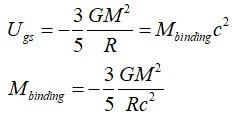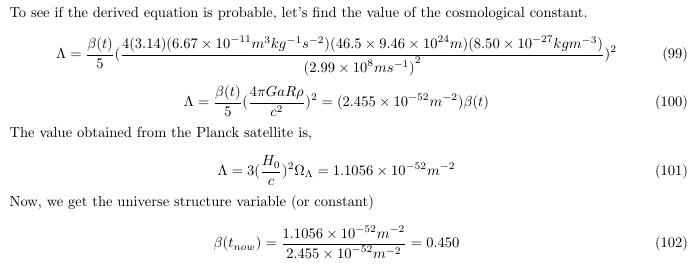# Dark energy is gravitational self-energy or the gravitational action of the gravitational field

#### 7icarus7

The source of dark energy is gravitational self-energy or the gravitational action of the gravitational field!

1. Gravitational potential energy is negative energy

1) Alan Guth said
The energy of a gravitational field is negative!
The positive energy of the false vacuum was compensated by the negative energy of gravity.
2) Stephen Hawking also said
The matter in the universe is made out of positive energy. However, the matter is all attracting itself by gravity. Two pieces of matter that are close to each other have less energy than the same two pieces a long way apart, because you have to expend energy to separate them against the gravitational force that is pulling them together. Thus, in a sense, the gravitational field has negative energy. In the case of a universe that is approximately uniform in space, one can show that this negative gravitational energy exactly cancels the positive energy represented by the matter. So the total energy of the universe is zero.
Both said that gravitational potential energy is negative energy and is the true energy that can cancel positive mass energy.

3) Negative energy (mass) density in standard cosmology
From the second Friedmann equation or acceleration equation,

(1/R)(d^2R/dt^2)=-(4πG/3)(ρ+3P)

In standard cosmology, it is explained by introducing an entity that has a positive mass density but exerts a negative pressure.

p + 3P = p + 3(-p) = - 2p

However, If we rearrange the dark energy term, the final result is a negative mass density of -2p.
In the standard cosmology, accelerated expansion is impossible without negative mass density. It is just that the negative mass density term is called negative pressure, so it is not recognized.

4) Gravitational binding energy and mass defect
https://en.wikipedia.org/wiki/Gravitational binding energy
Two bodies, placed at the distance R from each other and reciprocally not moving, exert a gravitational force on a third body slightly smaller when R is small. This can be seen as a negative mass component of the system, equal, for uniformly spherical solutions, to:5) Since gravitational self-energy is proportional to -M^2/R, The greater the mass, the greater the gravitational self-energy ratio
In the dimensional analysis of energy, E has kg(m/s)^2, so all energy can be expressed in the form of (mass) x (speed)^2. So, E=Mc^2 holds true for all kinds of energy. "-M_gs" is the equivalent mass of gravitational self-energy. It is a negative equivalent mass term.

Earth's -M_gs = (- 4.17x10^-10)M_Earth
Sun's -M_gs = (- 1.27x10^-4)M_Sun
Black hole's -M_gs = (- 0.3)M_Black-hole

For example, in the case of a black hole,
-M_gs=-(3/5)(GM^2)/(Rc^2)=-(3/5)(GM^2)/((2GM/c^2)c^2)=-(3/10)M=-0.3M_Black-hole

What about the universe with more mass?

2. In the universe, if we calculate the gravitational self-energy or total gravitational potential energy
The universe is almost flat, and its mass density is also very low. Thus, Newtonian mechanics approximation can be applied.
Since the particle horizon is the range of interaction, if we find the Mass energy (Mc^2) and Gravitational self-energy ((-M_gs)c^2) values at each particle horizon, Mass energy is an attractive component, and the equivalent mass of gravitational self-energy is a repulsive component. Critical density value p_c = 8.50 x 10^-27[kgm^-3] was used.

At particle horizon R=16.7Gly, (-M_gs)c^2 = (-0.39M)c^2 : |(-M_gs)c^2| < (Mc^2) : Decelerated expansion period
At particle horizon R=26.2Gly, (-M_gs)c^2 = (-1.00M)c^2 : |(-M_gs)c^2| = (Mc^2) : Inflection point (About 5-7 billion years ago, consistent with standard cosmology.)
At particle horizon R=46.5Gly, (-M_gs)c^2 = (-3.04M)c^2 : |(-M_gs)c^2| > (Mc^2) : Accelerated expansion period

Even in the universe, gravitational potential energy (or gravitational action of the gravitational field) must be considered.
And, in fact, if we calculate the value, since gravitational self-energy is larger than mass energy, so the universe has accelerated expansion.
Gravitational self-energy accounts for decelerated expansion, inflection point, and accelerated expansion.

3. New Friedmann's equations and the dark energy term from the gravitational self-energy modelFinding the dark energy term with this model is similar to the standard cosmology value.
Gravitational potential energy is a concept that has already existed, and does not assume a bizarre existence that has a positive inertial mass and exerts a negative pressure. There are no problems like fine tuning or CCC problem.

In standard cosmology, the energy density of dark energy is a constant. However, in the gravitational self-energy model, dark energy density is a variable.
The dark energy (or cosmological constant term) is a function of time. Thus, this model can be verified.

# Dark energy is the Gravitational Potential Energy or Gravitational Self-Energy
https://www.researchgate.net/publication/360096238

Last edited:

#### 7icarus7

*Background description
Why do we need gravitational potential energy or gravitational field energy?
1) The fundamental principle of general relativity states that “all energy is a source of gravity”. However, the field equation created by Einstein did not fully realize this principle

The energy of the gravitational field must also function as a gravitational source.
Einstein was also aware of this, and for over two years, beginning in 1913, he worked to formulate a field equation that included the energy-momentum of the gravitational field. However, because it was difficult to define the energy of the gravitational field in general relativity, Einstein could not complete the field equation including the gravitational action of the gravitational field.
So, the singularity problem and the dark energy problem came into existence.

2) As the mass increases, the ratio of negative gravitational potential energy to mass energy increases
-M_gs = Equivalent mass of total gravitational potential energy (gravitational self energy) of an object
Earth's -M_gs = (- 4.17 x 10^-10)M_Earth
Sun's -M_gs = (- 1.27 x 10^-4)M_Sun
Black hole's -M_gs = (- 3 x 10^-1)M_Black-hole
What about the universe with more mass?

1. Gravitation and Spacetime (Book) : 25~29PDifferent forms of energy as source of gravity : Gravitational energy in Earth

If we want to discover whether gravity gravitates, we must examine the behavior of large masses,of planetary size, with significant and calculable amounts of gravitational self-energy. Treating the Earth as a continuous, classical mass distribution (with no gravitational self-energy in the elementary, subatomic particles), we find that its gravitational self-energy is about 4.6×10^−10 times its rest-mass energy. The gravitational self-energy of the Moon is smaller, only about 0.2 × 10^−10 times its rest-mass energy.
2. Explanation of GRAVITY PROBE B team
Do gravitational fields produce their own gravity?

A gravitational field contains energy just like electromagnetic fields do. This energy also produces its own gravity, and this means that unlike all other fields, gravity can interact with itself and is not 'neutral'. The energy locked up in the gravitational field of the earth is about equal to the mass of Mount Everest, so that for most applications, you do not have to worry about this 'self-interaction' of gravity when you calculate how other bodies move in the earth's gravitational field.
3. Wikipedia, Gravitational binding energy
Two bodies, placed at the distance R from each other and reciprocally not moving, exert a gravitational force on a third body slightly smaller when R is small. This can be seen as a negative mass component of the system, equal, for uniformly spherical solutions, to:

M_binding=-(3/5)(GM^2)/(Rc^2)

For example, the fact that Earth is a gravitationally-bound sphere of its current size costs 2.49421×1015 kg of mass (roughly one fourth the mass of Phobos – see above for the same value in Joules), and if its atoms were sparse over an arbitrarily large volume the Earth would weigh its current mass plus 2.49421×1015 kg kilograms (and its gravitational pull over a third body would be accordingly stronger).
4. Gravitation and Spacetime (Book)
In writing the field equation (48) we have assumed that the quantity T^{\mu \nu } is the energy-momentum tensor of matter. In order to obtain a linear field equation we have left out the effect of the gravitational field upon itself. Because of this omission, our linear field equation has several (related) defects:

(1) According to (48) matter acts on the gravitational field (changes the fields), but there is no mutual action of gravitational fields on matter; that is, the gravitational field can acquire energy-momentum from matter, but nevertheless the energy-momentum of matter is conserved ({\partial _\nu }{T^{\mu \nu }} = 0). This is an inconsistency.
(2) Gravitational energy does not act as source of gravitation, in contradiction to the principle of equivalence. Thus, although Eq. (48) may be a fair approximation in the case of weak gravitational fields, it cannot be an exact equation.
The obvious way to correct for our sin of omission is to include the energy-momentum tensor of the gravitational field in T^{\mu \nu }. This means that we take for the quantity T^{\mu \nu } the total energy-momentum tensor of matter plus gravitation:

T^{\mu \nu }} = T_{(m)}^{\mu \nu } + {t^{\mu \nu }}
5. Problem with Einstein's field equation

The basis of modern cosmology is Einstein's 1915 field equation. However, this field equation has a singularity problem inside the black hole. In other words, the field equation created by Einstein is likely to be incomplete.

The fundamental principle of general relativity states that “all energy is a source of gravity”. However, the field equation created by Einstein did not fully realize this principle.

The energy of the gravitational field must also function as a gravitational source.
Einstein was also aware of this, and for over two years, beginning in 1913, he worked to formulate a field equation that included the energy of the gravitational field. However, because it was difficult to define the energy of the gravitational field in general relativity, Einstein could not complete the field equation including the gravitational action of the gravitational field. So, without the energy-momentum tensor of the gravitational field, the 1915 field equation came out.

Because it was difficult to define the energy-momentum tensor of the gravitational field in general relativity, in this paper, based on the corresponding principle that general relativity is approximated by Newtonian mechanics in a weak field, I approach the gravitational action of the gravitational field as an approximate way. And I prove that this method suggests sufficiently meaningful results in cosmology.

There are Landau–Lifshitz pseudotensor and Einstein pseudotensor methods for describing the energy-momentum of the gravitational field, but it does not seem to have formed a consensus among all people yet. In addition, it seems that solution of singularity through these pseudo-tensor methods or cosmological application methods have not yet been proposed. As described in this paper, the energy of the gravitational field is likely a source of dark energy, so if you can, try it from these pseudo-tensors or from a new field equation with the energy-momentum tensor of the gravitational field.

#Dark Energy is Gravitational Potential Energy or Gravitational Self Energy
#On the solution of the strong gravitational field the solution of the Singularity problem the origin of Dark energy and Dark matter

Last edited: